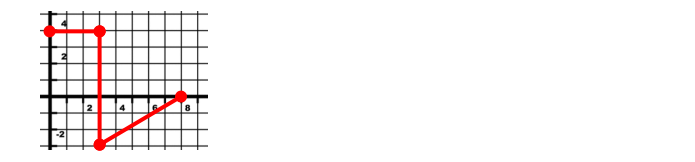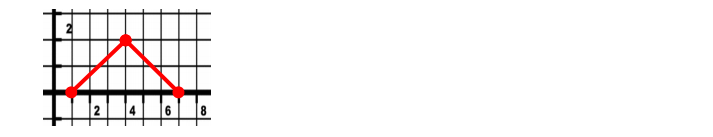Ch 11: Momentum & ImpulseWorksheetSee all chapters
 Ch 01: Intro to Physics; Units 1hr & 22mins 0% complete WorksheetDownload the video lesson worksheet Ch 02: 1D Motion / Kinematics 4hrs & 13mins 0% complete WorksheetDownload the video lesson worksheet Ch 03: Vectors 2hrs & 2mins 0% complete WorksheetDownload the video lesson worksheet Ch 04: 2D Kinematics 2hrs 0% complete WorksheetDownload the video lesson worksheet Ch 05: Projectile Motion 2hrs & 57mins 0% complete WorksheetDownload the video lesson worksheet Ch 06: Intro to Forces (Dynamics) 3hrs & 2mins 0% complete WorksheetDownload the video lesson worksheet Ch 07: Friction, Inclines, Systems 2hrs & 27mins 0% complete WorksheetDownload the video lesson worksheet Ch 08: Centripetal Forces & Gravitation 2hrs & 53mins 0% complete WorksheetDownload the video lesson worksheet Ch 09: Work & Energy 3hrs & 56mins 0% complete WorksheetDownload the video lesson worksheet Ch 10: Conservation of Energy 7hrs & 4mins 0% complete WorksheetDownload the video lesson worksheet Ch 11: Momentum & Impulse 5hrs & 35mins 0% complete WorksheetDownload the video lesson worksheet Ch 12: Rotational Kinematics 3hrs & 4mins 0% complete WorksheetDownload the video lesson worksheet Ch 13: Rotational Inertia & Energy 7hrs & 7mins 0% complete WorksheetDownload the video lesson worksheet Ch 14: Torque & Rotational Dynamics 2hrs & 9mins 0% complete WorksheetDownload the video lesson worksheet Ch 15: Rotational Equilibrium 4hrs & 10mins 0% complete WorksheetDownload the video lesson worksheet Ch 16: Angular Momentum 3hrs & 6mins 0% complete WorksheetDownload the video lesson worksheet Ch 17: Periodic Motion 2hrs & 17mins 0% complete WorksheetDownload the video lesson worksheet Ch 19: Waves & Sound 3hrs & 25mins 0% complete WorksheetDownload the video lesson worksheet Ch 20: Fluid Mechanics 4hrs & 39mins 0% complete WorksheetDownload the video lesson worksheet Ch 21: Heat and Temperature 4hrs & 9mins 0% complete WorksheetDownload the video lesson worksheet Ch 22: Kinetic Theory of Ideal Gasses 1hr & 40mins 0% complete WorksheetDownload the video lesson worksheet Ch 23: The First Law of Thermodynamics 2hrs & 15mins 0% complete WorksheetDownload the video lesson worksheet Ch 24: The Second Law of Thermodynamics 4hrs & 56mins 0% complete WorksheetDownload the video lesson worksheet Ch 25: Electric Force & Field; Gauss' Law 3hrs & 32mins 0% complete WorksheetDownload the video lesson worksheet Ch 26: Electric Potential 1hr & 55mins 0% complete WorksheetDownload the video lesson worksheet Ch 27: Capacitors & Dielectrics 2hrs & 2mins 0% complete WorksheetDownload the video lesson worksheet Ch 28: Resistors & DC Circuits 3hrs & 20mins 0% complete WorksheetDownload the video lesson worksheet Ch 29: Magnetic Fields and Forces 2hrs & 35mins 0% complete WorksheetDownload the video lesson worksheet Ch 30: Sources of Magnetic Field 2hrs & 30mins 0% complete WorksheetDownload the video lesson worksheet Ch 31: Induction and Inductance 3hrs & 38mins 0% complete WorksheetDownload the video lesson worksheet Ch 32: Alternating Current 2hrs & 37mins 0% complete WorksheetDownload the video lesson worksheet Ch 33: Electromagnetic Waves 1hr & 12mins 0% complete WorksheetDownload the video lesson worksheet Ch 34: Geometric Optics 3hrs 0% complete WorksheetDownload the video lesson worksheet Ch 35: Wave Optics 1hr & 15mins 0% complete WorksheetDownload the video lesson worksheet Ch 37: Special Relativity 2hrs & 10mins 0% complete WorksheetDownload the video lesson worksheet Ch 38: Particle-Wave Duality Not available yet Ch 39: Atomic Structure Not available yet Ch 40: Nuclear Physics Not available yet Ch 41: Quantum Mechanics Not available yet

# Impulse with Variable Forces

See all sections
Sections
Intro to Momentum
Intro to Impulse
Impulse with Variable Forces
Intro to Conservation of Momentum
Push-Away Problems
Adding Mass to a Moving System
How to Identify the Type of Collision
Inelastic Collisions
2D Collisions
Newton's Second Law and Momentum
Momentum & Impulse in 2D
Push-Away Problems With Energy
Elastic Collisions
Collisions & Motion (Momentum & Energy)
Collisions with Springs
Intro to Center of Mass

Concept #1: Impulse with Variable Forces

Transcript

Hey guys. So, now we're going to talk about impulse and momentum problems when forces are not variable, let's check it out. So, so far everything we've done you might have noticed, talked about an object average force, average force, and you may remember that an average force is also a constant force and that's because constant forces are much simpler than variable forces but the problem is when you have collisions forces are not constant, when you have collisions forces are variable, they're changing up. So, to make things easier, we said? Well, let's just then talk about the average force, when there's a collision, typically what happens is something like this, object is moving and then it hits right here and the force goes up and then it goes down like this, okay? So, this is force over time. So, what do we do? We talked about the average force, okay? Think about, when like a tennis ball hits a wall over here, right? So, as soon as it starts touching the wall starts pushing on it, the more that the ball compresses the more sort of a surface area has to be pushed against and then it starts sort of loosening up and if you just press your arm like slowly, your hand slowly like this, in your arm you can tell it hurts more and more and then as it goes away it stops hurting, okay? So, it looks, most collisions will look something like this. Now, when we have problems where forces are variable, which is new. So, we're just now introducing this, the total impulse will be the area under the force time graph, if you've done um if you've done work so far you may remember that work is the area under a force times position graph, this is similar, J is the area under force time graph, okay? So, it's the same concept. Again, if you've covered work and energy great, if you haven't that's fine, don't worry about that, you'll get to it.

So, got a few examples here, some practice problems for us to try, I have 5 kilogram object initially at rest, so the mass is 5, the initial velocity is 0 and it's acted upon by a variable force according to the diagram below. So, a force time graph, that's how it's always going to be, force is measured in Newtons and time is measured in seconds. Says, calculate the total impulse delivered to the object. So, total impulse is given by this equation here, Alright, we can use any one of these forms to do it but if you look around, we actually don't have the, we don't have enough information to do this basically, and this is actually not applicable in this case because this only works for a average force or a constant force average and constant mean average forces are constant forces. So, a more generic way to write this would be like this? Well, the force is not constant. So, instead it's going to be the area of f, t graph, cool? So, it's the area of this graph here, since, I have a graph that looks like this, it goes up then it goes. Now, we have to segment is graphed into two areas. So, I'm going to split this up, this is area 1 and this is area 2. Alright, so this is going to be a1 plus a2, this is really simple, these are right triangles, so the area of any triangle is just b, h over 2, base times height divided 2 and then I'm going to do this, this is for the first one, and I'm going to do this for the second one b, h 2 over 2, okay? So, very straightforward, if you plug in the numbers the base here is 123, that's a 3 right there, the base here is from 3 to 7. So, it's 1, 2, 3, 4, 5, 8 actually. So, this base here is 5 and the height for both of them obviously is 4, so this is 4, 3 over 2, this is 5 or 4, 5 over 2 and if you combine this you get 16, okay? The unit is obviously n, s because it's joules, I mean, not joules, impulse cool, what about the object's final speed? So, how do we find v final? Well, now we can use part of that impulse equation to do this because impulse is area or Delta p or m, V final, V initial, this still works, right? So, we can do that then we can, v initial is 0, we can use this to find v final, impulse is 16, the mass is 5 and then V final is right there. So, v final is 16 over 5, its 3.2 meters per second, very straightforward, the only new thing here is calculating the area, you probably get some really simple area and that's it. So, I got a practice problem, I want you to give it a try, just remember that this area is a little bit different than this area, hopefully you can get it, let's try.

Practice: The force shown in the force-time graph below acts on a 4-kg object. If the object has final speed (at t = 8 s) of 3 m/s, calculate its initial speed (at t = 0 s).Practice: A 650-g basketball is dropped from 3.0 m. When it bounces on a hard surface, it experiences a variable force as shown below (the y-axis is in kN, the x-axis is in ms). What is the ball’s speed immediately after bouncing? (Use g=10 m/s2.)